##### Interest - Simple, Annual, Continous and Discount Factors
Mathematics of converting interest rates from one basis to another
Key Terms:
• A discount factor is equal to NPV (today)/Nominal Value (in the future). It is the factor by which you multiply the future cash flow in order to arrive at the Net Present Value.
• The Continuous Discount Rate is the rate you get if you assume compounding takes place continually. It"s easiest to think of (although its not technically true) as the daily compounding rate. In other words, if you wonder what daily compounded rate would be equivalent to a certain end result, then that"s the continually compounded rate.
• An Annual Equivalent Rate is the equivalent interest rate if interest were charged annually in arrears and compounded. It turns out that all interest rates have a period associated with them. (See Annual Equivalent Rates)
• A Simple Interest Rate is any interest rate that is applied "simply" to a time period. An AER is equal to a simple interest rate charged over 1 year. In our terminology a simple interest rate always has a period or payments per year associated with it. So you can say "a rate of x% simple interest charged quarterly is equivalent to an AER of y% which is also equivalent to a daily, or continuous rate, of z%".

To convert Simple Interest Rates to AER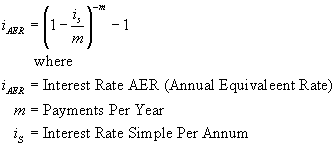This formula is used in the function SimpleToAER.

To convert AER to Simple InterestThis formula is used in the function AERToSimple.

To convert Simple Interest to ContinuousThis formula is used in the function SimpleToCont.

To Convert Continuous to Simple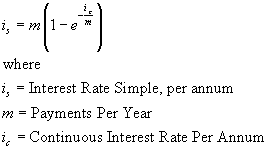This formula is used in the function ContToSimple.

To convert AER to Continuous: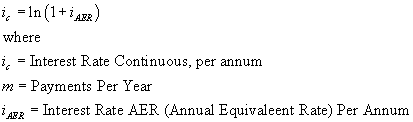This formula is used in the function AERToCont.

To convert Continuous to AER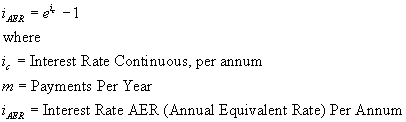This formula is used in the function ContToAER.

To convert an AER to a Discount Factor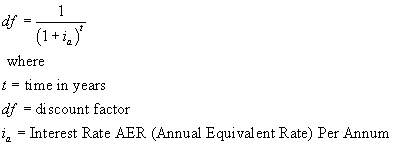This formula is used in the function AERToDF.

To convert a Discount Factor to an AER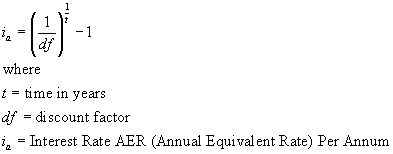This formula is used in the function DFToAER.

To convert a Continuous rate to a Discount Factor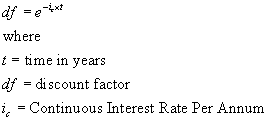This formula is used in the function ContToDF.

To convert a Discount Factor to a Continuous Rate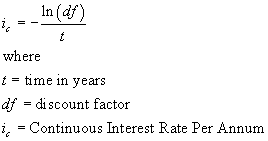This formula is used in the function DFToCont.

To convert a Simple rate to a Discount Factor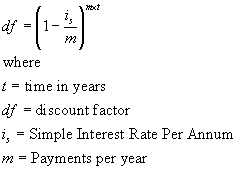This formula is used in the function SimpleToDF.

To convert a Discount Factor to a Simple RateThis formula is used in the function DFToSimple.

Business Functions Ltd, London, UK Website Design: Webpure